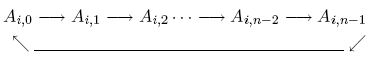Online JudgeProblem SetAuthorsOnline ContestsUser
Web Board
F.A.Qs
Statistical Charts
Problems
Submit Problem
Online Status
Prob.ID:
Register
Authors ranklist
Current Contest
Past Contests
Scheduled Contests
Award Contest
Register
Language:
Matrix
 Time Limit: 2000MS Memory Limit: 30000K Total Submissions: 4914 Accepted: 2601

Description

Given an n*n matrix A, whose entries Ai,j are integer numbers ( 0 <= i < n, 0 <= j < n ). An operation SHIFT at row i ( 0 <= i < n ) will move the integers in the row one position right, and the rightmost integer will wrap around to the leftmost column.You can do the SHIFT operation at arbitrary row, and as many times as you like. Your task is to minimize
max0<=j< n{Cj|Cj=Σ0<=i< nAi,j}

Input

The input consists of several test cases. The first line of each test case contains an integer n. Each of the following n lines contains n integers, indicating the matrix A. The input is terminated by a single line with an integer −1. You may assume that 1 <= n <= 7 and |Ai,j| < 104.

Output

For each test case, print a line containing the minimum value of the maximum of column sums.

Sample Input

```2
4 6
3 7
3
1 2 3
4 5 6
7 8 9
-1```

Sample Output

```11
15```

Source

[Submit]   [Go Back]   [Status]   [Discuss]Home PageGo BackTo top